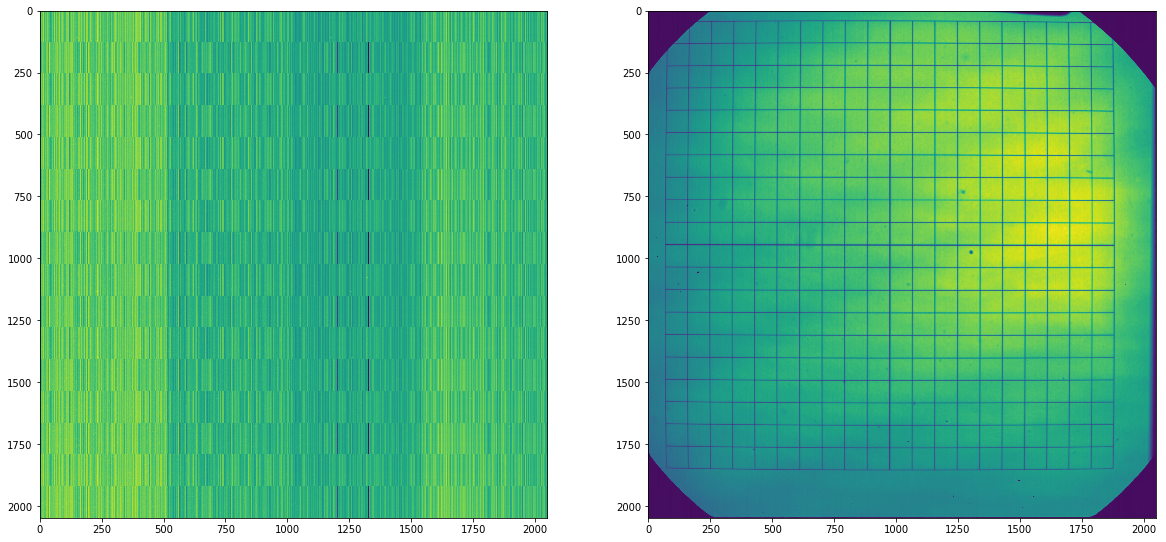# How to use the rectangular grid to estimate the geometrical distortion in the image plane of a telescope

## Using grid lines but improving the corner detection¶

In :
```import numpy as np
import pyfits
import matplotlib.pyplot as plt
import skimage
from skimage.feature import blob_dog, blob_doh, blob_log, canny
from skimage.color import rgb2gray
from skimage.feature import corner_harris, corner_subpix, corner_peaks
from skimage.segmentation import slic
from skimage.filters import sobel
from scipy.signal import convolve2d
from scipy.ndimage import gaussian_filter
from skimage import measure
from scipy.optimize import curve_fit
import matplotlib.ticker as mtick
```
In :
```path = '/home/fatima/Desktop/solar_orbiter_project/codes/targets/distortion/'
path_paper = '/home/fatima/Desktop/solar_orbiter_project/codes/notebooks/distortion/images_paper/'
#grid = pyfits.getdata(path+'solo_L0_0000015224_20170411T152003.fits')
grid = pyfits.getdata(path+'calibration_lines.fits')
dark = pyfits.getdata(path+'dark.fits')
#grid = grid/dark

#points = points[402:1813,52:1816] #circles
#grid = grid[135:1759,168:1792]
```
In :
```fig=plt.figure(figsize=(20,10))
ax1.imshow(dark)
ax2.imshow(grid)
```
Out:
`<matplotlib.image.AxesImage at 0x7fe91ccbc910>`In :
```edge = sobel(grid)
```
In :
```#hdu = pyfits.PrimaryHDU(edge)
#hdu.writeto('edge.fits')
```
In :
```fig=plt.figure(figsize=(25,12))
plt.imshow(edge)
```
Out:
`<matplotlib.image.AxesImage at 0x7fbebe4c5b50>`